Jesse Parete

•Analysis of Graph - Increasing/Decreasing - Concavity

Activity

Jesse Parete

•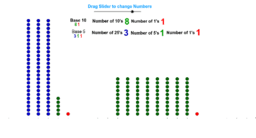Graphs of NUMBERS Under 100 - base 10 & 5

Activity

Jesse Parete

•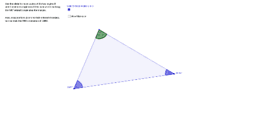Sum of the Interior Angles of a Triangle

Activity

Jesse Parete

•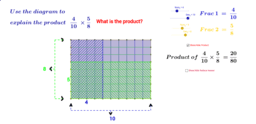Dynamic Diagram for the Product of Two Fractions

Activity

Jesse Parete

•Linear Equations and Inequalities - Systems

Activity

Jesse Parete

•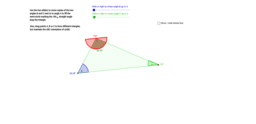The Sum of the Angles of a Triangle

Activity

Jesse Parete

•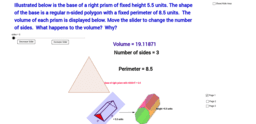5-E_Volume

Activity

Jesse Parete

•5-E_AreaRectFixedPerimeter20Ft

Activity

Jesse Parete

•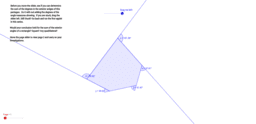2nd Investigation-Sum of Exterior Angles for polygons

Activity

Jesse Parete

•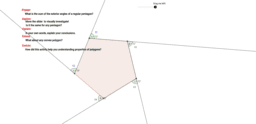Sum of Exterior Angles for polygons-1st Investigation

Activity

Jesse Parete

•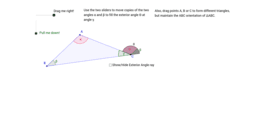Exterior Angle of a Triangle

Activity

Jesse Parete

•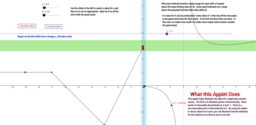Continuity and Limits

Activity

Jesse Parete

•Activity

Jesse Parete

•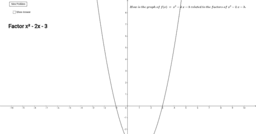Activity

Jesse Parete

•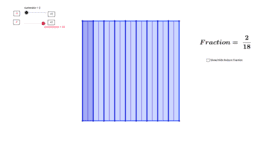Simple Fractions

Activity

Jesse Parete

•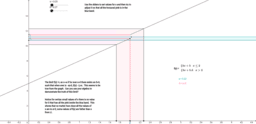Demo of Delta-Epsilon definition for a discontinous F.

Activity

Jesse Parete

•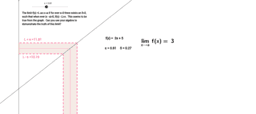Delta-Epsilon definition illustrated

Activity

Jesse Parete

•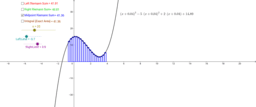Riemann Sums

Activity

Jesse Parete

•Unit Circle and Trigonometry

Activity

Jesse Parete

•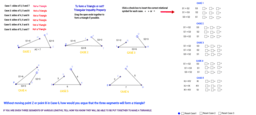Triangle Inequality

Activity

Jesse Parete

•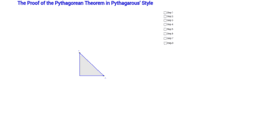The Proof of the Pythagorean Theorem in Pythagarous' Style

Activity

Jesse Parete

•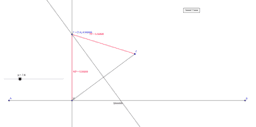Parabola by Locus Definition

Activity

Jesse Parete

•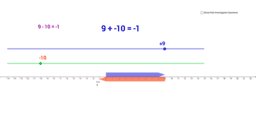•